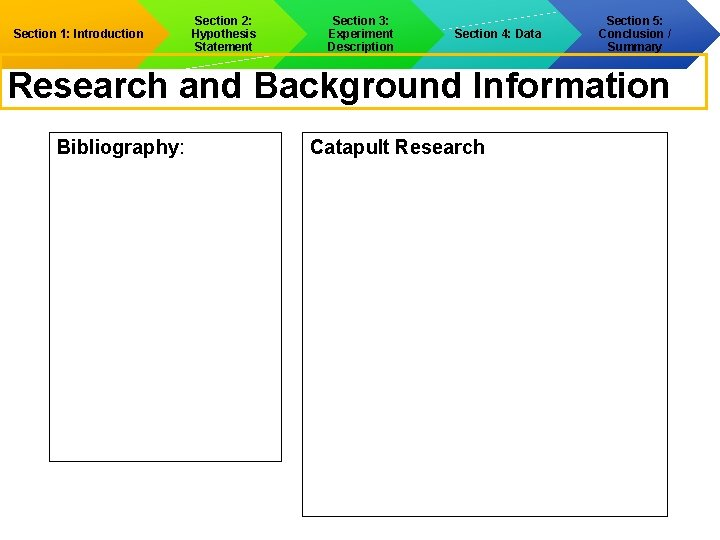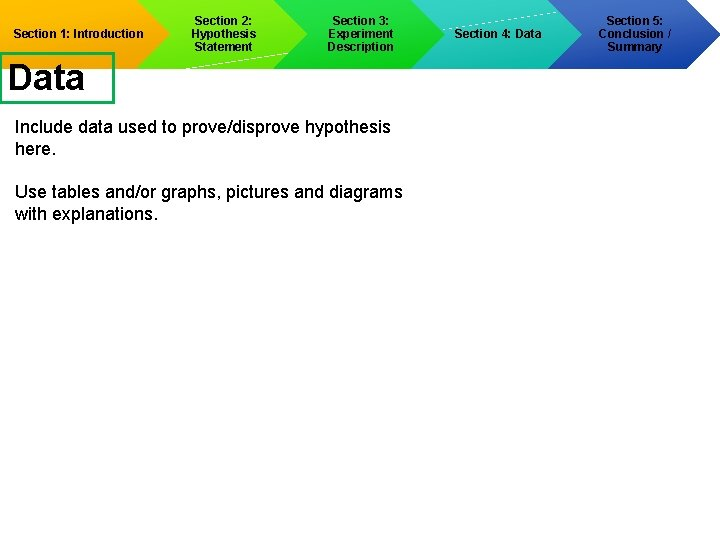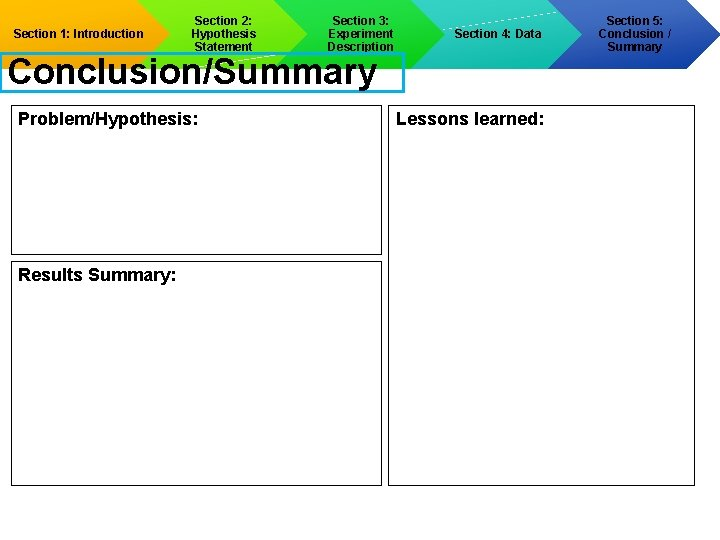# Section 1 Introduction Section 2 Hypothesis Statement Section

• Slides: 8Section 1: Introduction Section 2: Hypothesis Statement Section 3: Experiment Description Section 4: Data Section 5: Conclusion / Summary Replace with project title Life Science Angelina County Science & Tech Fair 2018 Replace with participant names and grade level Replace with School Name Replace with Teacher NameSection 1: Introduction Section 2: Hypothesis Statement Section 3: Experiment Description Section 4: Data Introduction Problem Statement: Goal: ________: Section 5: Conclusion / SummarySection 1: Introduction Section 2: Hypothesis Statement Section 3: Experiment Description Section 4: Data Section 5: Conclusion / Summary Research and Background Information Bibliography: Catapult ResearchSection 1: Introduction Section 2: Hypothesis Statement Project Progress Journal Date/Time Section 3: Experiment Description Summary/Accomplishments Section 4: Data Section 5: Conclusion / SummarySection 1: Introduction Section 2: Hypothesis Statement Section 3: Experiment Description Section 4: Data Hypothesis Proposed Expectation of Results: Variables: Section 5: Conclusion / SummarySection 1: Introduction Section 2: Hypothesis Statement Section 3: Experiment Description Section 4: Data Experimental Description Procedure: Material Quantity Cost Source Section 5: Conclusion / SummarySection 1: Introduction Section 2: Hypothesis Statement Section 3: Experiment Description Data Include data used to prove/disprove hypothesis here. Use tables and/or graphs, pictures and diagrams with explanations. Section 4: Data Section 5: Conclusion / SummarySection 1: Introduction Section 2: Hypothesis Statement Section 3: Experiment Description Section 4: Data Conclusion/Summary Problem/Hypothesis: Results Summary: Lessons learned: Section 5: Conclusion / Summary